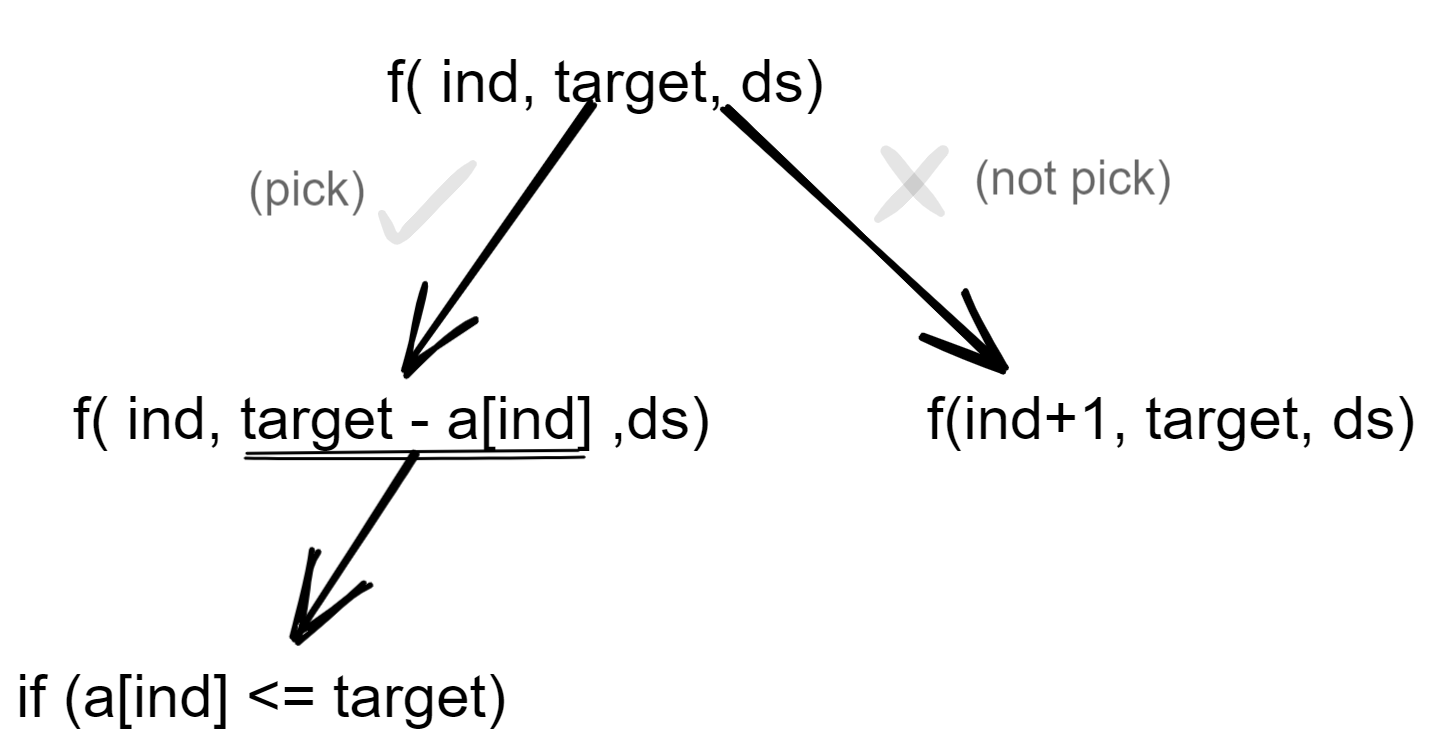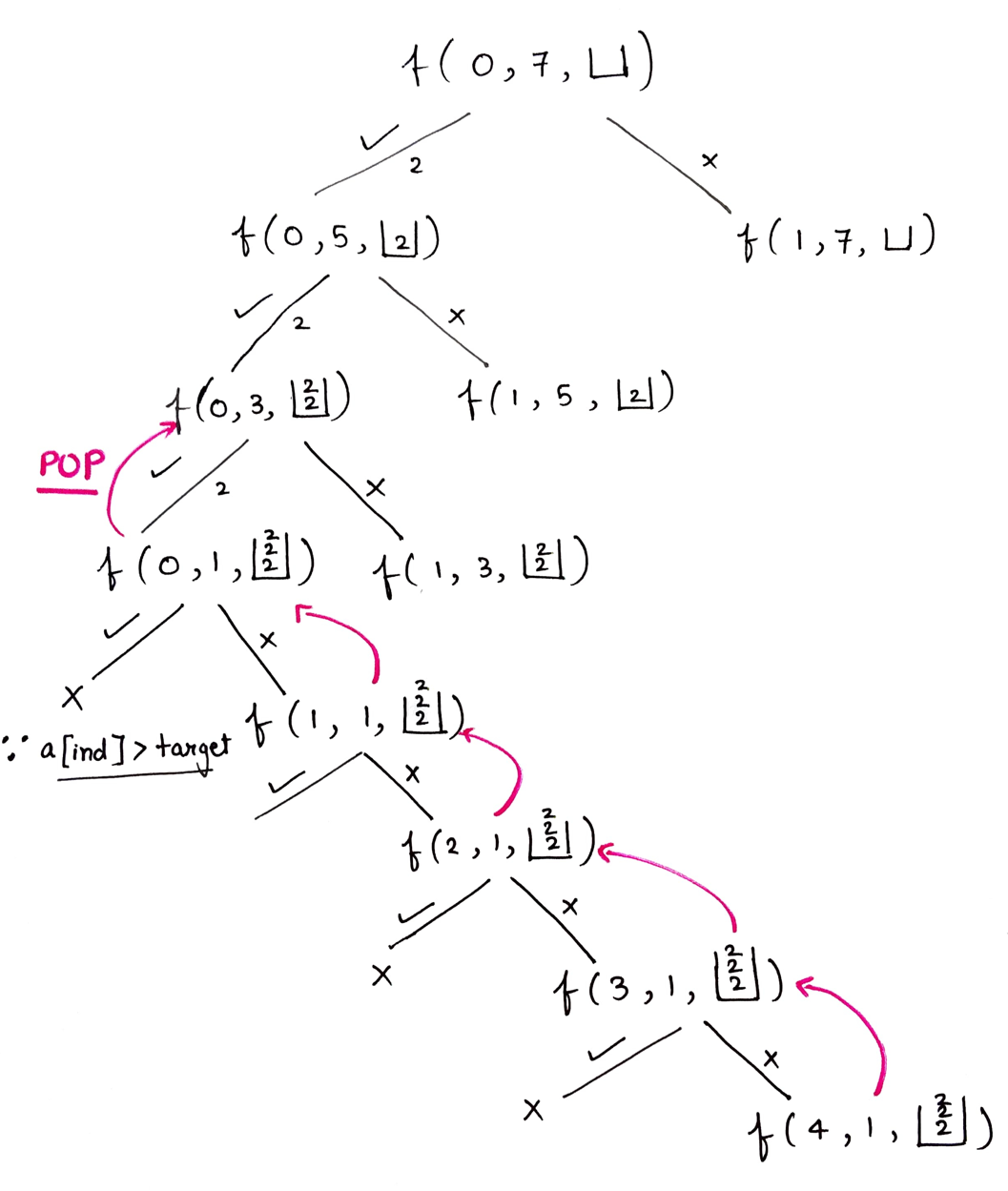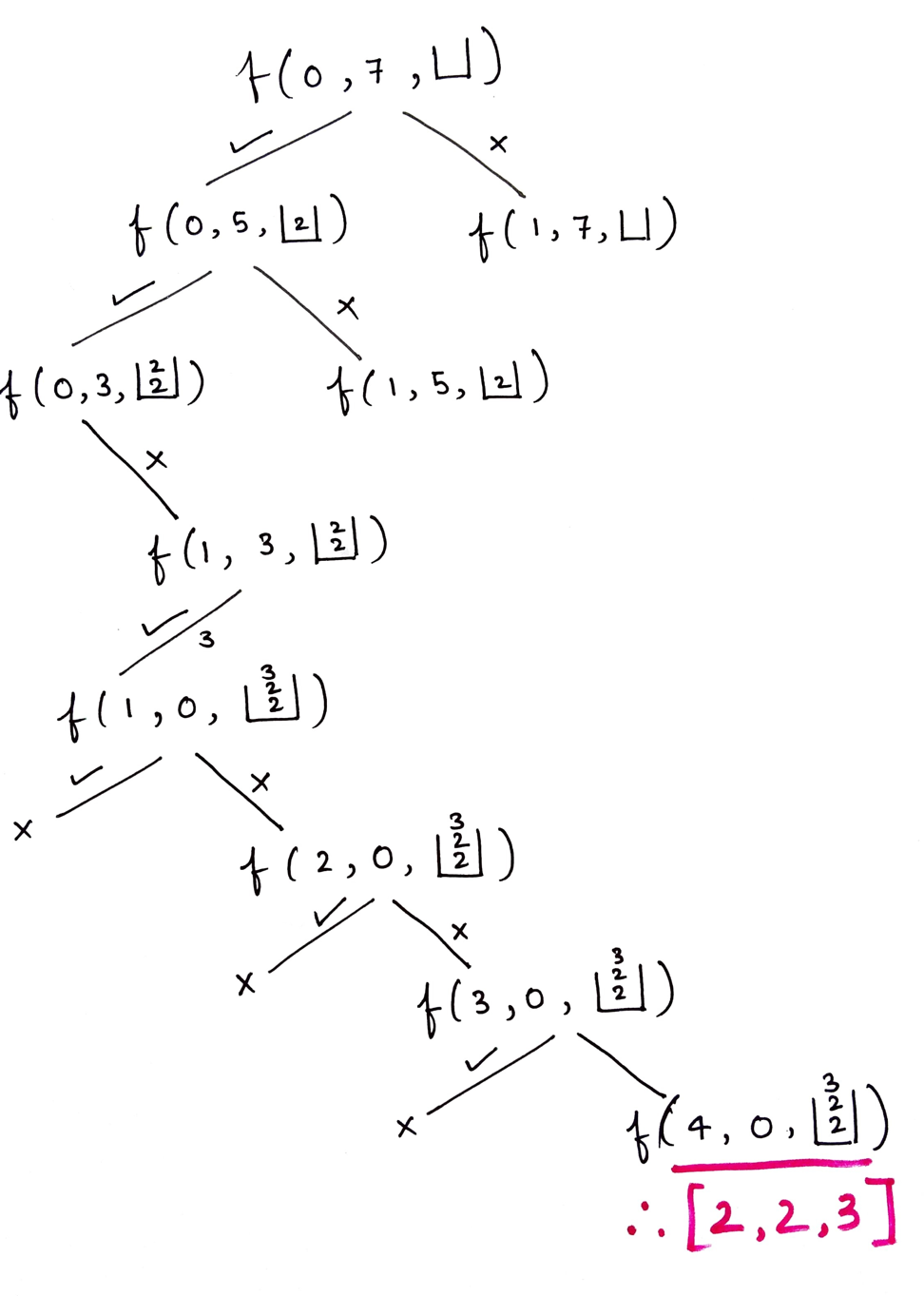# Combination Sum – 1

Problem Statement:

Given an array of distinct integers and a target, you have to return the list of all unique combinations where the chosen numbers sum to target. You may return the combinations in any order.

The same number may be chosen from the given array an unlimited number of times. Two combinations are unique if the frequency of at least one of the chosen numbers is different.

It is guaranteed that the number of unique combinations that sum up to target is less than 150 combinations for the given input.

Examples:

```Example 1:

Input: array = [2,3,6,7], target = 7

Output: [[2,2,3],]

Explanation: 2 and 3 are candidates, and 2 + 2 + 3 = 7. Note that 2 can be used multiple times.
7 is a candidate, and 7 = 7.
These are the only two combinations.

Example 2:

Input: array = , target = 1

Output: []

Explaination: No combination is possible.```

### Solution

Disclaimer: Don’t jump directly to the solution, try it out yourself first.

Solution: Recursion

Intuition:

For questions like printing combinations or subsequences, the first thing that should strike your mind is recursion.

How to think recursively?

Whenever the problem is related to picking up elements from an array to form a combination, start thinking about the “pick and non-pick” approach.

Approach

Defining recursive function:Initially, the index will be 0, target as given and the data structure(vector or list) will be empty

Now there are 2 options viz to pick or not pick the current index element.

If you pick the element, again come back at the same index as multiple occurrences of the same element is possible so the target reduces to target – arr[index] (where target -arr[index]>=0)and also insert the current element into the data structure.

If you decide not to pick the current element, move on to the next index and the target value stays as it is. Also, the current element is not inserted into the data structure.

While backtracking makes sure to pop the last element as shown in the recursion tree below.

Keep on repeating this process while index < size of the array for a particular recursion call.

You can also stop the recursion when the target value is 0, but here a generalized version without adding too many conditions is considered.

Using this approach, we can get all the combinations.

Base condition

If index== size of array and  target == 0 include the combination in our answer

Diagrammatic representation for Example 1:

Case 1:Case 2:Code:

## C++ Code

``````#include<bits/stdc++.h>

using namespace std;
class Solution {
public:
void findCombination(int ind, int target, vector < int > & arr, vector < vector < int >> & ans, vector < int > & ds) {
if (ind == arr.size()) {
if (target == 0) {
ans.push_back(ds);
}
return;
}
// pick up the element
if (arr[ind] <= target) {
ds.push_back(arr[ind]);
findCombination(ind, target - arr[ind], arr, ans, ds);
ds.pop_back();
}

findCombination(ind + 1, target, arr, ans, ds);

}
public:
vector < vector < int >> combinationSum(vector < int > & candidates, int target) {
vector < vector < int >> ans;
vector < int > ds;
findCombination(0, target, candidates, ans, ds);
return ans;
}
};
int main() {
Solution obj;
vector < int > v {2,3,6,7};
int target = 7;

vector < vector < int >> ans = obj.combinationSum(v, target);
cout << "Combinations are: " << endl;
for (int i = 0; i < ans.size(); i++) {
for (int j = 0; j < ans[i].size(); j++)
cout << ans[i][j] << " ";
cout << endl;
}
}``````

Output:

Combinations are:
2 2 3
7

Time Complexity: O(2^t * k) where t is the target, k is the average length

Reason: Assume if you were not allowed to pick a single element multiple times, every element will have a couple of options: pick or not pick which is 2^n different recursion calls, also assuming that the average length of every combination generated is k. (to put length k data structure into another data structure)

Why not (2^n) but (2^t) (where n is the size of an array)?

Assume that there is 1 and the target you want to reach is 10 so 10 times you can “pick or not pick” an element.

Space Complexity: O(k*x), k is the average length and x is the no. of combinations

## Java Code

``````import java.io.*;
import java.util.*;
class Solution {

private void findCombinations(int ind, int[] arr, int target, List < List < Integer >> ans, List < Integer > ds) {
if (ind == arr.length) {
if (target == 0) {
}
return;
}

if (arr[ind] <= target) {
findCombinations(ind, arr, target - arr[ind], ans, ds);
ds.remove(ds.size() - 1);
}
findCombinations(ind + 1, arr, target, ans, ds);
}
public List < List < Integer >> combinationSum(int[] candidates, int target) {
List < List < Integer >> ans = new ArrayList < > ();
findCombinations(0, candidates, target, ans, new ArrayList < > ());
return ans;
}
}
public class Main {
public static void main(String[] args) {
int arr[] = {2,3,6,7};
int target = 7;
Solution sol = new Solution();
List < List < Integer >> ls = sol.combinationSum(arr, target);
System.out.println("Combinations are: ");
for (int i = 0; i < ls.size(); i++) {
for (int j = 0; j < ls.get(i).size(); j++) {
System.out.print(ls.get(i).get(j) + " ");
}
System.out.println();
}
}
}``````

Output:

Combinations are:
2 2 3
7

Time Complexity: O(2^t * k) where t is the target, k is the average length

Reason: Assume if you were not allowed to pick a single element multiple times, every element will have a couple of options: pick or not pick which is 2^n different recursion calls, also assuming that the average length of every combination generated is k. (to put length k data structure into another data structure)

Why not (2^n) but (2^t) (where n is the size of an array)?

Assume that there is 1 and the target you want to reach is 10 so 10 times you can “pick or not pick” an element.

Space Complexity: O(k*x), k is the average length and x is the no. of combinations